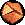What is the Radius of Convergence?

If you take math in your first year of college, they teach you about infinite series and the radius of convergence. An infinite series is a polynomial in a variable X with and infinite number of terms ...

f(X) = K0 + K1 X + K2 X2 + K3 X3 + ...
In particular, let's consider the series with all of the Ki are one,
f(X) = 1 + X + X2 + X3 + ...
If we pick X=1/2, we have to add up smaller and smaller terms 1 + 1/2 + 1/4 + 1/8 + 1/16 + ... forever, with each number half the size of the previous. Each number takes you half the remaining distance to two: the sum is said to converge to two.

Adding up an infinite number of numbers doesn't always give a sensible answer. Suppose all the Ki are one, but X=2: we then get the sum 1 + 2 + 4 + 8 + 16 ... which clearly becomes infinite. (Sometimes we say the sum converges to infinity, but usually we say it diverges). If we set X=-2, we get an even worse problem: 1 - 2 + 4 - 8 + 16 ... If we start on the left and add up the numbers in order, we get 1, then -1, then 3, then -5, then 11 ... we flip-flop back and forth between larger and larger positive and negative numbers.

If you work at it, you can convince yourself that for X bigger than one, or for X smaller than minus one, the infinite sum doesn't make sense (it diverges). For X smaller than one and bigger than minus one, the sum can be done. The radius of convergence for this function is one.

Four more things we should mention.

1. It's called a radius of convergence because mathematicians like to plug in complex numbers, like X = U + i V, where i * i = -1. They can show that the series converges inside a circle U2 + V2 = R2, and diverges outside the circle.
2. If you're clever, you can figure out that our sum (with all the Ki=1), when it converges, always gives 1/(1-X). An infinite series represents a function. (Taylor's theorm tells us how to get the series from the function.) Other infinite series Ki can be used to make other functions.
3. This sum 1/(1-X) does become big when X gets close to X=1. On the other hand, there doesn't seem to be anything wrong at X=-1. The convergence of the infinite series at X=-1 is spoiled because of a problem far away at X=1, which happens to be at the same distance from zero! The radius of convergence is usually the distance to the nearest point where the function blows up or gets weird.
4. There is a simple way to calculate the radius of convergence of a series Ki (the ratio test). The series can't possibly converge unless the terms eventually get smaller and smaller. If we insist that |Kn+1 Xn+1| be smaller than |Kn Xn|, that can happen only if |X| is smaller than |Kn/Kn+1|. The radius of convergence of an infinite series is (basically) the value of |Kn/Kn+1| for large n.Statistical Mechanics: Entropy, Order Parameters, and Complexity, now available at Oxford University Press (USA, Europe).